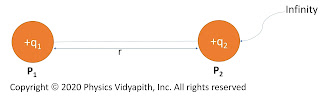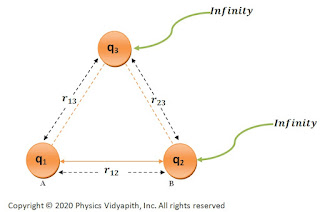### The electric potential energy of a system of Charges

The Potential Energy of a system of two-point like charges→

When the system of two charged particles is configured, in which one charge is at rest of position and another is brought from infinity to near the first charge then the work done acquire by this charged particle is stored in the form of electric potential energy between these charges.

Derivation→

Let us consider, If two charge $q_{1}$ and $q_{2}$ in which one charge $q_{1}$ is at the rest of the position at point $P_{1}$ and another charge $q_{2}$ is brought from infinity to a point $P$ to configure the system then the electric potential at point $P_{2}$ due to charge particle $q_{1}$ →Electric potential energy between two like charges
$V=\frac{1}{4\pi\epsilon_{0}}\frac{q_{1}}{r}$

Where $r$ is the distance between the point $P_{1}$ and Point $P_{2}$

Here, Charge $q_{2}$ is moved in from infinity to point $P_{2}$ then the work required is →

$W=V q_{2}$

$W= \frac{1}{4\pi\epsilon_{0}}\frac{q_{1}q_{2}}{r}$

Since the electric potential at infinity is zero so work- done will also be zero. So total work done from infinity to a point $P$ will be stored in the form of electric potential energy.

$U=W$

 $U= \frac{1}{4\pi\epsilon_{0}}\frac{q_{1}q_{2}}{r}$

The electric potential energy of a system of three-point-like charges→

To obtain the potential energy of a system of three charges. First, obtain the work done between any two charges and then obtain the different work done for both those charges from the third charge and then the total work done will be equal to electric potential energy.

Let us consider a system is made up of three charges $q_{1}$, $q_{2}$ and $q_{3}$ which placed at point $P_{1}$,$P_{2}$ and $P_{3}$. Now the work done between two charges $q_{1}$ and $q_{2}$ isElectric potential energy between three like charges
$W_{1}=\frac{1}{4\pi\epsilon_{0}}\frac{q_{1}q_{2}}{r_{12}}$

Now, the charge $q_{3}$ is brought from infinity to the point $P_{3}$. Work has to be done against the forces exerted by $q_{1}$ and $q_{2}$ Therefore, The work done between charges of $q_{2}$ and $q_{3}$

$W_{2}=\frac{1}{4\pi\epsilon_{0}} \frac{q_{2}q_{3}}{r_{23}}$

Now, The work done between charges $q_{1}$ and $q_{3}$

$W_{3}=\frac{1}{4\pi\epsilon_{0}} \frac{q_{1}q_{3}}{r_{13}}$

The total work done to make a system of three charges:

$W=W_{1}+W_{2}++W_{3}$

Now substitute the value of $W_{1}$, $W_{2}$and $W_{2}$ in above equation i.e.

$W=\frac{1}{4\pi\epsilon_{0}} \left[\frac{q_{1}q_{2}}{r_{12}}+\frac{q_{1}q_{3}}{r_{13}}+\frac{q_{2}q_{3}}{r_{23}}\right]$

This work is stored in the form of electric potential energy in the system.

$U=W$

 $U=\frac{1}{4\pi\epsilon_{0}} \left[\frac{q_{1}q_{2}}{r_{12}}+ \frac{q_{1}q_{3}}{r_{13}}+\frac{q_{2}q_{3}}{r_{23}}\right]$

Similarly, the Potential energy of a system of N point system i.e.

 $U=\frac{1}{4\pi\epsilon_{0}} \sum_{i=1}^{N}\sum_{j=1}^{N} \frac{q_{i}q_{j}}{r_{ij}}$

Here $i\neq j$